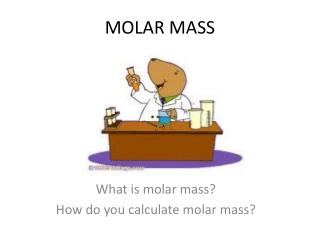DownloadDownload PresentationMOLAR MASS

# MOLAR MASS

Télécharger la présentation## MOLAR MASS

- - - - - - - - - - - - - - - - - - - - - - - - - - - E N D - - - - - - - - - - - - - - - - - - - - - - - - - - -
##### Presentation Transcript

1. MOLAR MASS What is molar mass? How do you calculate molar mass?

2. WHAT IS MOLAR MASS? • mass in grams of 1 mole of the element • Molar Mass = ___grams____ (MM) 1 Mol Synonyms: molecular weight, gram formula unit

3. Use Periodic Table to determine MM! • Let’s examine periodic table! • If I had one mole of _____ it’s molar mass would be _______ g per 1 mol.

4. Carbon MM= 12.0 g / mol • Atomic Hydrogen MM= 1.0 g / mol • Mercury MM= 200.6 g / mol • Iron MM=55.8 g / mol • Sulfur MM= 32.1 g / mol

5. How many particles do these elements contain? Particles: Atoms Formula Units Molecules

6. How do you calculate molar mass of compounds? SO3 • EX. Sulfur Trioxide Chemical Formula = _____________ • How many total atoms make up one molecule? • Atoms = _____________ • S: ___ x ______ = • O: ___ x ______ = _____ + • The molar mass of sulfur trioxide = _______ grams per ________ 1 + 3 = 4 1 32.1 32.1 48.0 16.0 3 80.1 80.1 1 mol

7. Your Turn PCl3 • EX. Phosphorus Trichloride Chemical Formula = __________ • How many total atoms make up one molecule? Atoms = ________ • P: ___ x ______ = • Cl: ___ x ______ = _________ + • Sodium Hydrogen Carbonate NaHCO3 1+3=4 1 31.0 31.0 3 35.5 106.5 137.5 grams per 1 mol MM = 84.0 g / mol

8. Representative Particles molecules • Covalent Compounds are made up of _____. • Ionic Compounds are made up of _________. • Elementsare made up of _____________. • Use your Road Map! Formula units atoms

9. Conversions Using Molar Mass • Book pg. 298 • Ex. #1 Write the chemical formula for aluminum oxide _________ • What is given? Trying to find? • ______________ x _______________ = g of Al2O3 GIVEN Al2O3 9.45 moles g of Al2O3 9.45 moles Al2O3 102.9 grams Al2O3 964 1 Mole Al2O3 Al: 2 x 27.0 = 54.0 g O: 3 x 16.0 = 48.0 g + 102.0 g / 1 mol

10. Molar Volume Conversions • At STP (Standard Temp and Pressure): • 1 mole of gas = 22.4 L = 6.02 x 1023 particles

11. 1 Mole of ANY GAS at STP!

12. Sample Problem 10.7 Use our Mole Road Map! • Sample Problem 10.8 • REMEMBER! Density = mass = grams Volume Liter • HINT: If given density, first find the molar mass (MM) = gramsper mol